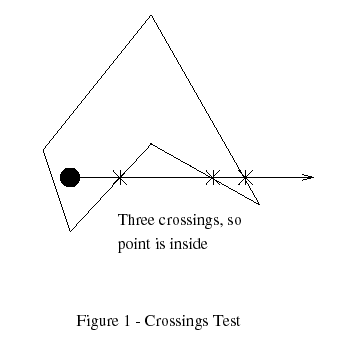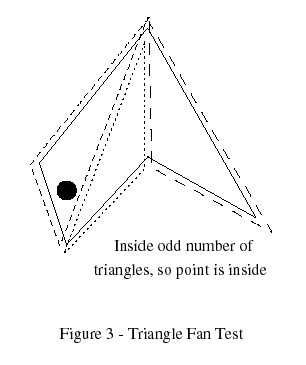# Point in Polygon

## Ray Casting Algorithm​

The algorithm is based on a simple observation that if a point moves along a ray from infinity to the probe point and if it crosses the boundary of a polygon, possibly several times, then it alternately goes from the outside to inside, then from the inside to the outside, etc. As a result, after every two "border crossings" the moving point goes outside## Triangle Tests​

In Graphics Gems, Didier Badouel (Badoeul 1990) presents a method of testing points against convex polygons. The polygon is treated as a fan of triangles emanating from one vertex and the point is tested against each triangle by computing its barycentric coordinates.### Optimization​

Bounding Box

Point in polygon algorithms benefit from having a bounding box around polygons with many edges. The point is first tested against this box before the full polygon test is performed; if the box is missed, so is the polygon. Most statistics generated in this Gem assume this bounding box test was already passed successfully.

Bins Method

One method to speed up testing is to classify the edges by their Y components and then test only those edges with a chance of intersecting the test point's X+ test ray. The bounding box surrounding the polygon is split into a number of horizontal bins and the parts of the edges in a bin are kept in a list, sorted by the minimum X component. The maximum X of the edge is also saved, along with a flag noting whether the edge fully crosses the bin's Y bounds. In addition, the minimum and maximum X components of all the edges in each bin are recorded.

When a point is to be classified, the proper bin is retrieved and if the point is outside the X bounds, it must be outside the polygon. Else, the list is traversed and the edges tested against the point. Essentially, a modified crossings test is done, with additional speed coming from the sorted order and from the storage of the "fully crosses" condition